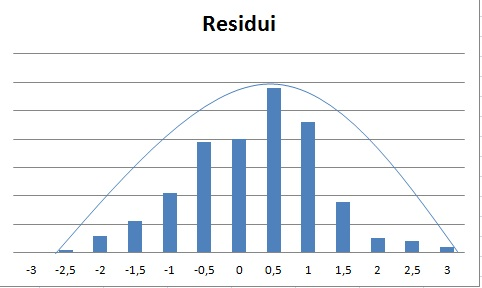# Does this histogram of residuals indicate that the residuals are effectively random?

I am studying a univariate and discrete time series. I know that residuals should be effectively random and have a good fit, and should have a bell shape.

Does the plot below suggest that the residuals are effectively random?• Welcome to the site, @Marco. I have no idea what you are asking. Can you clarify your question? May 13, 2013 at 21:51
• thanks. I am studying a time series with a classical approach. I want that someone describe this graphic and tell me if this graphic describe the residuals effectively random. May 13, 2013 at 22:19
• What's the y (vertical) axis on the graph represent? May 13, 2013 at 23:00
• It is good to look at how the residuals are distributed. However, this histogram tells you very little about their apparent "randomness." For that, you need to compare the residuals to other data you have, including the dependent variable and any other variables that might not have been involved in the fitting. You want the residuals to look independent of all other variables.
– whuber
May 14, 2013 at 17:46
• In addition to whuber's helpful comments, one way to try to rule out non-random patterns in residuals is to create a scatterplot of the residuals (on the vertical axis) against either the dependent variable or the predicted values of it (on the horizontal axis). Ideally one would see no systematic increase or decrease in the mean or the variation as one moved from left to right. May 15, 2013 at 0:32

Welcome to CrossValidated, Marco!

If I understood you correctly, you are using Least Squares Estimator (LSE) for your regression problem. In order to operate effectively, LSE indeed requires normally distributed residuals. A good way to check this is to have a look at so-called Q-Q plot: you draw the quantiles of your obtained residuals versus theoretical normal quantiles. If you see something as a line in the Q-Q plot - you are done - the assumption of normality is fulfilled.

But I want to encourage you to be careful, you also have to check other assumptions required for LSE: independence of the residuals and homoscedasticity.

Hope it will help!

• Linear regression requires normal errors??
– kirk
May 14, 2013 at 14:16
• @kirk , linear regression itself not, but Least Squares estimator for linear regression is equivalent to the Maximum Likelihood estimator with gaussian errors. That's why it is often assumed that errors should be normally distributed. And as I get from the question (reference to bell-curve), this is exactly what is asked to check. May 15, 2013 at 18:30

First the curve you have drawn is not the bell you're looking for. Your "bell" should be more like this:Your histogram-drawn-as-a-bar-chart (yikes! Excel encourages terrible things) looks reasonably close to that.

However, histograms are not a very good way to check for normality of residuals.

As discussed here, on occasions - and depending on your choices for where the histogram bars go, the same set of values might look as different as these:Just to repeat - that's two different histograms of the same numbers. Kernel density estimates and better still, QQ plots (at least once you learn how to read them) are significantly more informative. If you must use histograms, use plenty of bins and do more than one.